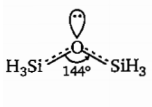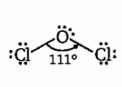The incorrect statement regarding $\mathrm{O}{\left({\mathrm{SiH}}_{3}\right)}_{2}$ and ${\mathrm{OCl}}_{2}$ molecule is/are:

(a) The strength of back bonding is more in $\mathrm{O}{\left({\mathrm{SiH}}_{3}\right)}_{2}$ molecule than ${\mathrm{OCl}}_{2}$ molecule

(b) $\mathrm{Si}—\mathrm{O}—\mathrm{Si}$ bond angle in $\mathrm{O}{\left({\mathrm{SiH}}_{3}\right)}_{2}$ is greater than $\mathrm{Cl}—\mathrm{O}—\mathrm{Cl}$ bond angle in ${\mathrm{OCl}}_{2}$

(c) The nature of back bond in both molecules is $2{\mathrm{p}}_{\sigma }‐3{\mathrm{d}}_{\mathrm{\pi }}$

(d) Hybridisation of central $\mathrm{O}‐\mathrm{atom}$ in both molecules is same

Concept Videos :-

#7 | Hybridization in case of Carbon| Intro
#8 | Hybridization in case of Carbon| sp3
#9 | Hybridization in case of Carbon| sp2
#10 | Hybridization in case of Carbon| sp
#11 | Hybridization in case of other Molecules

Concept Questions :-

Hybridisation

(d)Hybridisation of O-atom ${\mathrm{Sp}}^{2}$ due to                        Hybridisation of O-atom remains ${\mathrm{Sp}}^{3}$,

more extent of $\left(2{\mathrm{p}}_{\sigma }-3{\mathrm{d}}_{\mathrm{\pi }}\right)$ back                              because of less effective $\left(2{\mathrm{p}}_{\sigma }-3{\mathrm{d}}_{\mathrm{\pi }}\right)$

bonding                                                                back bonding due to d-orbital resonance

Difficulty Level:

• 24%
• 32%
• 16%
• 30%